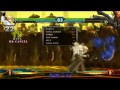## Media Library - KOF XIII: King combo tutorial - King, the Muay Thai Bouncer.

KOF XIII: King combo tutorial - King, the Muay Thai Bouncer.KOF XIII: King combo tutorial - King, the Muay Thai Bouncer. (3 min 50 sec)
Uploaded on 11-07-2011 at 12:42 PM by RonanN1

King is a really good well balanced character in this game. She has tons of combo potential (maybe to many possibilities) and doesnt seem to unfair as far as damage goes. She has damaging combos with EX moves, dream-cancels and in Maxmode, but nothing is really to powerful.
Most of her long combos that start outside of the corner and end in the corner are distance specific. For example combos 14, 15, 22 and 23. If you dont have the right distance, they will always mess up. I would avoid them all unless you are always aware of your position.

Her (B) tornado kick is great if you want juggle after in the corner. Messing around with dream cancel trap-shot into Tornado kick, gives you some good options for further combos and is easy to use.

One thing I must point out and its important to her game. If you want to combo trap shot after DF+D, you must delay executing the trap-shot for a split second. If you do it to fast, it will always miss. So remember that.

1 - jump+D, std+D, df+D, qcf+D
2 - jump+D, std+D, df+D, hcb+D
3 - dwn+B, dwn+B, std+B, dp+B
4 - jump+D, std+D (1st hit), qcfx2+A
5 - jump+D, std+D, df+D, qcfx2+D
6 - jump+D, std+D, df+D, dp+B, qcfx2+A+C
7 - jump+D, std+D, df+D, qcf+D, qcfx2+D
8 - jump+D, std+D, df+D, qcf+B+D, qcfx2+D
9 - jump+D, std+D, df+D, qcf+B+D, qcfx2+A+C
10 - jump+D, std+D, df+D, qcbx2+B+D
11 - jump+D, std+D, df+D, hcb+D, qcbx2+B+D
12 - jump+D, std+D, df+D, qcf+B+D, hcb+D, qcbx2+B+D
13 - CORNER: jump+D, std+D, df+D, qcf+B+D, dp+B, qcf+B+D, qcf+B, qcfx2+A+C
14 - SP: jump+D, std+D, df+D, dp+B, qcfx2+B, qcfx2+D
15 - SP: jump+D, std+D, df+D, dp+B, qcfx2+B, hcb+D, qcf+B+D, qcfx2+B, hcb+D
16 - CORNER: jump+D, std+D, df+D, hcb+D, qcf+B+D, dp+B, hcb+B, qcfx2+B, qcfx2+A+C
17 - CORNER: jump+D, std+D, df+D, hcb+B, dp+B, hcb+B, dp+B, hcb+B, qcfx2+B, qcfx2+A+C
18 - CORNER: jump+C, qcf+B+D, hcb+D, qcf+B+D, qcfx2+B, hcb+D, qcf+B+D, qcfx2+B, hcb+D
19 - jump+D, std+D, df+D, B+C Cancel, std+D, df+D, qcbx2+B+D
20 - jump+D, std+D, df+D, B+C Cancel, std+D, df+D, dp+B, hcb+B, qcfx2+B, qcbx2+B+D
21 - jump+D, std+D, df+D, B+C Cancel, std+D, df+D, dp+B, qcfx2+A+C, qcbx2+B+D
22 - jump+D, std+D, df+D, B+C Cancel, std+D, df+D, dp+B, hcb+B, qcfx2+B, hcb+D, qcf+B+D, dp+B, hcb+B, dp+B, hcb+B, qcfx2+B, qcfx2+A+C
23 - jump+D, std+D, df+D, B+C Cancel, std+D, df+D, dp+B, hcb+B, qcfx2+B, hcb+D, qcf+B+D, qcfx2+B, hcb+D, qcf+B+D, qcfx2+D
24 - CORNER: jump+D, std+D, df+D, B+C Cancel, std+D, df+D, dp+B, hcb+B, dp+B, hcb+B, dp+B, hcb+B, dp+B, hcb+B, qcfx2+B, qcfx2+A+C
25 - CORNER: jump+D, std+D, df+D, B+C Cancel, std+D, df+D, dp+B, hcb+B, dp+B, hcb+B, qcfx2+A+C, qcbx2+B+D

Please subscribe and support if you'd like me to release more KOF XIII videos.

Tags: king, king of fighters 13, king of fighters xiii, kof 13, kof xiii

KOF XIII: King combo tutorial - King, the Muay Thai Bouncer.